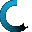Retrieving data ...
Please do not click anywhere else.

If you see no response after 2-3 minutes, then click on the [X] button in the upper right corner to close the window and retry the operation at a later time.
Interactive Multimedia Learning & Teaching Management System Suite## Course Contents

Course Name: Pre-Algebra (New) - 7th/8th Grade
• 1. Numbers Review
•  1.1 Whole Numbers
•  1.2 Integers
•  1.3 Positive and Negative Integers
•  1.4 Rational and Irrational Numbers
•  1.5 Decimal Numbers
•  1.6 Real Numbers
•  1.7 Prime Numbers
•  1.8 Percentages
• 2. Fractions and Operations with Fractions
•  2.1 Understanding Fractions
•  2.2 Equivalent Fractions
•  2.3 The Greatest Common Factor
•  2.4 The Least Common Multiple
•  2.5 The Least Common Denominator
•  2.6 Reducing Fractions
•  2.8 Adding Fractions and Whole Numbers
•  2.10 Multiplying Fractions
•  2.11 Multiplying Fractions and Whole Numbers
•  2.12 Subtracting Fractions
•  2.13 Subtracting Fractions and Whole Numbers
•  2.14 Subtracting Mixed Fractions
•  2.15 Dividing Fractions
•  2.16 Dividing Fractions and Whole Numbers
• 3. Real Numbers
•  3.1 Real Numbers
•  3.2 The Number Line
•  3.3 Comparing Real Numbers
•  3.4 Properties of Real Numbers
•  3.6 Subtracting Real Numbers
•  3.7 Multiplying Real Numbers
•  3.8 Dividing Real Numbers
•  3.9 Order of Operations
•  3.10 Mixed Operations with Real Numbers
• 4. Powers and Roots
•  4.1 Squares and Square Roots
•  4.2 Cubes and Cube Roots
•  4.3 Power of n and nth Roots
•  4.4 Powers of 10
•  4.5 Simplifying Square Roots
•  4.6 Simplifying Cube Roots
•  4.7 Scientific Notation
• 5. Exponents
•  5.1 Understanding Exponents
•  5.2 Basic Rules of Exponents
•  5.3 Laws of Exponents
•  5.4 Expressions Raised to a Power
•  5.5 Fractional Exponents
•  5.6 Negative Exponents
•  5.7 Multiplying & Dividing with Negative Exponents
•  5.8 Simplifying Exponential Expressions
•  5.9 Evaluating Exponential Expressions
• 6. Variables
•  6.1 Variables
•  6.2 Like and Unlike Terms
•  6.3 Canceling Variables
•  6.4 The Addition Operation with Variables
•  6.5 The Subtraction Operation with Variables
•  6.6 The Multiplication Operation with Variables
•  6.7 The Division Operation with Variables
• 7. Expressions, Equations and Formulas
•  7.1 Expressions and Equations
•  7.2 Writing Expressions and Equations
•  7.3 Writing Equations and Formulas
•  7.4 Problem Solving using Formulas
•  7.5 Comparing Real Number Expressions
•  7.6 Simplifying Expressions
•  7.7 Evaluating Expressions
•  7.9 Word Problems
• 8. The Coordinate Plane
•  8.1 The Coordinate Plane
•  8.2 Graphing Points on a Coordinate Plane
•  8.3 Graphing Linear Relations
•  8.4 Graphing Linear Relations using a t-Table
•  8.5 Making Predictions from Linear Patterns
• 9. Linear Equations and Formulas
•  9.1 Introduction to Linear Equations
•  9.2 Linear Equations with One Variable
•  9.3 Writing Linear Equations with One Variable
•  9.4 Solving Linear Equations with One Variable
•  9.5 Word Problems with Linear Equations
• 10. Linear Equations and Slope
•  10.1 Introduction to the Slope of a Straight Line
•  10.2 Finding Slope When Given 2 Points
•  10.3 Slope-Intercept Form of a Linear Equation
•  10.4 Writing Linear Equations When Given 2 points
•  10.5 Writing Linear Equations When Given Slope & a Point
•  10.6 Using Linear Equations to Find Parallel Lines
• 11. Quadratic Equations and Parabolas
•  11.3 Introduction to Parabolas
•  11.4 Identifying Equations and Graphing Parabolas
•  11.5 Identifying Linear and Non-linear Equations
• 12. Linear Inequalities
•  12.1 Linear Inequalities with One Variable
•  12.2 Solving Linear Inequalities with One Variable
•  12.3 Solving Linear Inequalities by Addition & Subtraction
•  12.4 Solving Linear Inequalities by Multiplication & Division
•  12.5 Solving Multi-Step Linear Inequalities
• 13. Polynomials
•  13.1 Monomials and Degree of Monomials
•  13.2 Multiplying Monomials
•  13.3 Polynomials and Degree of Polynomials
•  13.4 Adding and Subtracting Polynomials
•  13.5 Multiplying a Polynomial by a Monomial
•  13.6 Multiplying Polynomials
•  13.7 Special Binomial Products
• 14. Factoring
•  14.1 Factors and Prime Factorization
•  14.2 Greatest Common Factor of Monomials
•  14.3 Factoring Polynomials using the Distributive Property
•  14.4 Factoring Polynomials using Grouping
•  14.5 Factoring Trinomials: x2 + bx + c
•  14.6 Factoring Trinomials: ax2 + bx + c
•  14.7 Factoring the Difference of Squares
•  14.8 Simplifying Algebraic Ratios
•  14.9 Solving Algebraic Proportions
• 15. Pythagorean Theorem and Triangles
•  15.1 Triangles and their Properties
•  15.2 The Pythagorean Theorem
•  15.3 The Distance Formula
• 16. Introduction to Trigonometric Functions
•  16.1 Primary Trigonometric Functions
•  16.2 Secondary Trigonometric Functions
•  16.3 Measuring Angles in Degrees and Radians
•  16.4 Trigonometric Functions of Special Angles
•  16.5 Trigonometric Functions on the Coordinate Plane
•  16.6 Pythagorean Identities